## Solutions of Refraction of Light Lakhmir Singh Manjit Kaur MCQ, VSAQ, HOTS, and LAQ Pg No. 247 Class 10 Physics

Multiple Choice Questions (MCQs)-Pg-247

29. A spherical mirror and a spherical lens each have a focal length of, -15 cm. The mirror and the lens are likely to be:
A. Both concave.
B. Both convex.
C. The mirror is concave but the lens is convex.
D. The mirror is convex but the lens is concave.30. Linear magnification produced by a convex lens can be:
A. Less than 1 or more than 1
B. Less than 1 or equal to 1
C. More than 1 or equal to 1
D. Less than 1, equal to 1 or more than 1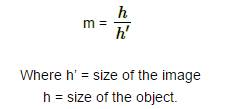31. Magnification produced by a concave lens is always:
A. More than 1
B. Equal to 1
C. Less than 1
D. More than 1 or less than 1

→ Magnification produce by concave mirror is less than 1 in two cases. As we know magnification is the enlargement of the object in form of image. And if the magnification is less then 1 then it is diminished. Also when object is placed at infinity or beyond centere of curvature then diminished image formed. That’s why is concave mirror there r two cases when magnification is less than 1.

32. In order to obtain a magnification of, -3 (minus 3) with a convex lens, the object should be placed:
A. Between optical center and F
B. Between F and 2F
C. At 2F
D. Beyond 2F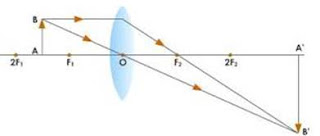33. A convex lens produces a magnification of +5. The object is placed;
A. At focus
B. Between ƒ and 2f
C. At Less than ƒ
D. Beyond 2 ƒ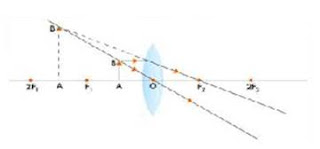34. If a Magnification of, -1 (minus 1) is obtained by using a converging lens, and then the object has to be placed:
A. Within ƒ
B. At 2 ƒ
C. Beyond 2 ƒ
D. At infinity35. To obtain a magnification of, -0.5 with a convex lens, the object should be placed:
A. At F
B. Between Optical centre and F
C. Between F and 2F
D. Beyond 2F36. An object is 0.09 m from a magnification lens and the image is formed 36 cm from the lens the magnification produced is:
A. 0.4
B. 1.4
C. 4.0
D. 4.5

→ Object distance = 0.09 m
image distance = 36 cm
m = v/u

37. To obtain a magnification of, -2 with a convex lens of focal length 10 cm, the object should be placed:
A. Between 5 cm and 10 cm
B. Between 10 cm and 20 cm
C. At 20 cm
D. Beyond 20 cm38. A convex lens of focal length 15 cm produces a magnification of +4. The object is placed:
A. At a distance of 15 cm
B. Between 15 cm and 30 cm
C. At less than 15 cm
D. Beyond 30 cm39. If a magnification of, -1 is to be obtained by using converging lens of focal length 12 cm, and then the object must be placed:
A. Within 12 cm
B. At 24 cm
C. T 6 cm
D. Beyond 24 cm40. In order to obtain a magnification of, -0.75 with a convex lens of focal length 8 cm, the object should be placed:
A. At less than 8 cm
B. Between 8 cm and 16 cm
C. Beyond 16 cm
D. At 16 cm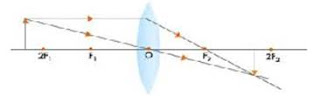Questions Based on High Order Thinking Skills (HOTS)-Pg-248

41. A student did an experiment with a convex lens. He put an object at different distances 25 cm, 30 cm, 40 cm, 60 cm and 120 cm from the lens. In each case he measured the distance of the image from the lens. His results were 100 cm, 24 cm, 60 cm, 30 cm and 40 cm, respectively. Unfortunately his results are written in wrong order.
(a) Rewrite the image distances in the correct order.
(b) What would be the image distance if the object distance was 90 cm?
(c) Which of the object distances gives the biggest image?
(d) What is the focal length of this lens?

→ (a) Since the focal length is a constant quantity, we have to pair the object distance(u) and the image distance (v) such that the focal length always comes out to be the same. From the above argument, we get the correct order of the image distance as  100 , 60 , 40 , 30 and 24. The reason being, as the object is carried far from a convex lens, the image is formed closer to the lens.
(b) Lens formula is given by:The image distance will be 25.7 cm if the object distance is 90 cm.
(c) The object distance of 25 gives the biggest image because at this position, the object is between f and 2f . We know that when an object is placed between f and 2f of a convex lens, we get a real, inverted and magnified image.
(d) The image of an object at 2f is formed at 2f. This means that the pair of u and v that is equal in value gives us the value of 2f, which is 40. Hence, the value of f (focal length) is 40/2
∴ f=20 cm.

42. A magnifying lens has a focal length of 100 mm. An object whose size is 16 mm is placed at some distance from the lens so that an image is formed at a distance of 25 cm in front of the lens.
(a) What is the distance between the object and the lens?
(b) Where should the object be placed if the image is to form at infinity?

→ (a) Given, focal length (f) = 100 mm
Size of object (h) = 16 mm
Image distance (v) = 25 cm = 250 mm
Lens formula is given by:The object is at a distance of 16.66 cm and in front of the mirror. The image is at a distance of
25 cm and in front of the mirror. Therefore, the distance between the object and the image is 25 cm-16.66 cm = 7.14 cm.
(b) The object should be placed at the focus for the image to be formed at infinity.

43. A lens forms a real image 3 cm high of an object 1 cm high. If the separation of object and image is 15 cm, find the focal length of the lens.44. An object 50 cm tall is placed on the principal axis of a convex lens. Its 20 cm tall image is armed on the screen placed at a distance of 10 cm from the lens. Calculate the focal length of the lens.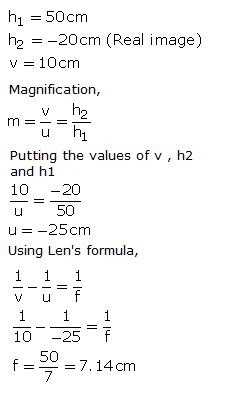1. If the image formed by a lens is always diminished and erect, what is the nature of the lens?

→ If the image formed by a lens is always diminished and erect, the nature of the lens is Concave lens.

2. Copy and complete the diagram below to show what happens to the rays of light when they pass through the concave lens: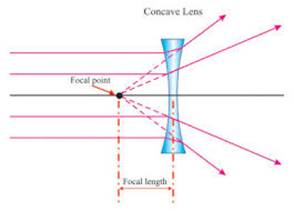3. Which type of lenses is?
(a) Thinner in the middle than at the edges?
(B) Thicker in the middle than at the edges?

→ (a) Concave lenses is Thinner in the middle than at the edges.(b) Convex lenses is Thicker in the middle than at the edges.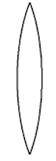4. A ray of light is going towards the focus of a concave lens. Draw a ray diagram to show the path of this ray of light after refraction through the lens.

→ Ray of light is going towards the focus of a concave lens. The ray diagram to show the path of this ray of light after refraction through the lens is as shown.5A. What type of images can a convex lens make?

→ Real and Virtual.

5B. What type of image is always made by a concave lens?

→ Virtual.

6. Take down this figure into your answer book and complete the path of the ray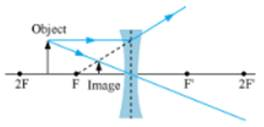7. Fill in the following blanks with suitable words:
(a) A ________ lens converges rays of light, whereas a ____ lens diverges rays of light.
(b) Lenses refract light to form images: a _______ lens can form ______ images, but a diverging lens forms only ______ images.

→ (a) convex; concave
(b) both real and virtual; virtual.

8. Things always look small on viewing through a lens. What is the nature of the lens?

→ Things always look small on viewing through Concave lens.

9. An object lies at a distance of 2f from a concave lens of focal length f. Draw a ray-diagram to illustrate the image formation.10. Show by drawing a ray-diagram that the image of an object formed by a concave lens is virtual, erect and diminished.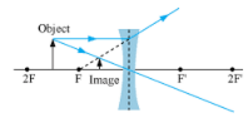11. Give the position, size and nature of image formed by a concave lens when the object is placed:
(a) Anywhere between optical center and infinity.
(b) At infinity.

→ (a) the position, size and nature of image formed by a concave lens when the object is placed placed anywhere between optical centre and infinity, is between optical centre and focus. It is diminished, virtual and erect.
(b) the position, size and nature of image formed by a concave lens when the object is placed at infinity, is at focus. It is highly diminished, virtual and erect.

12. Which type of lens is?
(a) A converging lens, and which is

→ (a) A convex lens is a converging lens because it converges a parallel beam of light rays passing through it at its focus.(b) A concave lens is a diverging lens because it diverges the parallel beam of rays passing through it.13. With the help of a diagram, explain why the image of an object viewed through a concave lens appears smaller and closer than the object.

→ The image of an object viewed through a concave lens appears smaller and closer than the object As shown by the diagram,14. How would a pencil look like if you saw it through?
(a) A concave lens, and
(b) A convex lens? (Assume the pencil is close to the lens). Is the image real or virtual?

→ (a) a pencil will look Smaller if we saw it through a concave lens.
(b) a pencil will look Bigger if we saw it through a convex lens; The image formed is Virtual.

15A. An object is placed 10 cm from a lens of focal length 5 cm. Draw the ray diagrams to show the formation of image if the lens is (i) converging, and (ii) diverging.

→ (i) the ray diagrams to show the formation of image if the lens is converging.(ii) the ray diagrams to show the formation of image if the lens is diverging.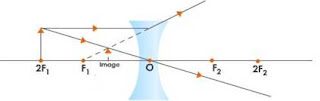15B. State one practical use each of convex mirror, concave mirror, convex lens and concave lens.

→ One practical use each of convex mirror, concave mirror, convex lens and concave lens is
Use of convex mirror: As rear-view mirror in vehicles
Use of concave mirror: As shaving mirrors
Use of convex lens: For making simple camera
Use of concave lens: As eye-lens in Galilean telescope

16A. Construct ray diagrams to illustrate the formation of a virtual image using
(i) a converging lens, and
(ii) A diverging lens.

→ (i) Formation of virtual image using a converging lens:(ii) Formation of virtual image using a diverging lens:16B. What is the difference between the two images formed above?

→ The virtual image formed by a converging lens is magnified whereas that formed by a diverging lens is diminished.

Multiple Choice Questions (MCQs)-Pg-252

17. A diverging lens is used in:
A. A magnifying glass
B. A car to see objects on rear side
C. Spectacles for the correction of short sight
D. A simple camera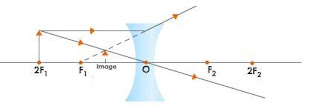18. When an object is kept at any distance in front of a concave lens, the image foiled is always:
A. Virtual, erect and magnified
B. Virtual inverted and diminished.
C. Virtual, erect and diminished
D. Virtual, erect and same size as object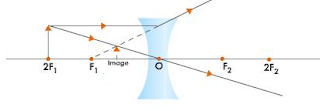19. When sunlight is concentrated on a piece of paper by a spherical mirror or lens, then a hole can be burnt in it. For doing this, the paper must be placed at the focus of:
A. Either a convex mirror or convex lens
B. either a concave mirror or concave lens
C. Either a concave mirror or convex lens
D. Either a convex mirror or concave lens

→ When sunlight is concentrated on a piece of paper by a spherical mirror or lens, then a hole can be burnt in it. For doing this, the paper must be placed at the focus of Concave mirror or convex lens.

20. A beam of parallel light rays is incident through the holes on one side of a box and emerges out through the holes on its opposite side as shown in the diagram below:
Which of the following could be inside the box?
A. A rectangular glass block
B. A concave lens
C. A convex lens
D. A glass prism21. A beam of light is incident through the holes on one side of a box and emerges out through the holes on its opposite side as shown in the following figure: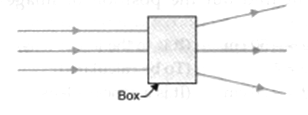The box contains:
A. A glass prism
B. A concave lens
C. A convex lens
D. A parallel-sided glass slab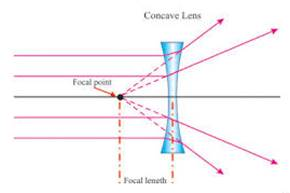22. Which of the following can form a virtual image which is always smaller than the object?
A. A plane mirror
B. a convex lens
C. A concave lens
D. a concave mirror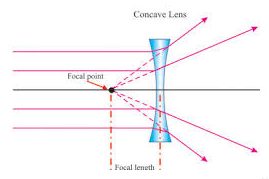Questions Based on High Order Thinking Skills (HOTS)-Pg-253

23. When an object is placed 20 cm in front of lens A, the image is real, inverted, magnified and formed at a great distance. When the same object is placed 20 cm in front of lens B, the image formed is real, inverted and same size as the object.
(a) What is the focal length of lens A?
(b) What is the focal length of lens B?
(c) What is the nature of lens A?
(d) What is the nature of lens B?

→ (a) The object is placed at focus, so f=20 cm of lens A.
(b) the object is placed at a distance twice the focal length, so f=10 cm of lens B.
(c) the nature of lens A is Convex lens (since image is real).
(d) the nature of lens B is Convex lens (since image is real).

24. When a fork is seen through lenses A and B one by one, it appears as shown in the diagrams. What is the nature of (i) lens A, and (ii) lens B? Give reason for your answer.→ (i) The nature of lens A is Concave lens as image appears diminished.
(ii) The nature of lens B is Convex lens as image appears enlarged.

25. What kind of lens can form?
(a) An inverted magnified image?
(b) An erect magnified image?
(c) An inverted diminished image?
(d) An erect diminished image?

→ (a) Convex lens can form An inverted magnified image.
(b) Convex lens can form An erect magnified image.
(c) Convex lens can form An inverted diminished image.
(d) Concave lens can form An erect diminished image.

1. The lens A produces a magnification of, - 0.6 whereas lens B produces a magnification of + 0.6.
(a) What is the nature of lens A?
(b) What is the nature of lens B?

→ (a) the nature of lens A is Convex lens
(b) the nature of lens B is Concave lens

2. A 50 cm tall object is at a very large distance from a diverging lens. A virtual, erect and diminished image of the object is formed at a distance of 30 cm in front of the lens. How much is the focal length of the lens?

→ When an object is placed at a very large distance from a diverging lens, then image is formed at the focus of the lens.
Therefore, the focal length of the lens is 30 cm.

3. An object is placed at a distance of 4 cm from a concave lens of focal length 8 cm. Find the position and nature of the image.4. A concave lens of focal length 15 cm forms an image 10 cm from the lens. How far is the object placed from the lens? Draw the ray-diagram.Here object distance = 30 cm
Focal length = 15 cm
Image distance = 10 cm.

5. An object 60 cm from a lens gives a virtual image at a distance of 20 cm in front of the lens. What is the focal length of the lens? Is the lens converging or diverging? Give reasons for your answer.

→ u = -60 cm
v = -20 cm
Using lens formula on solving, we get
Focal length = -30 cm
The lens is diverging as the focal length is negative.

6. A concave lens of 20 cm focal length has an object kept at a distance of 60 cm in front of the mirror. Compute the image distance.

→ Focal length, f = -20 cm
Object distance, u = -60cm
Using lens formula,
Image distance = -15 cm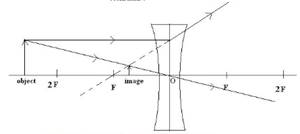7. A concave lens has focal length 20 cm. At what distance should the object from the lens be placed so that it forms an image at 10 cm from the lens?8. Calculate the image distance for an object of height 12 mm at a distance of 0.20 m from a concave lens of focal length 0.30 m?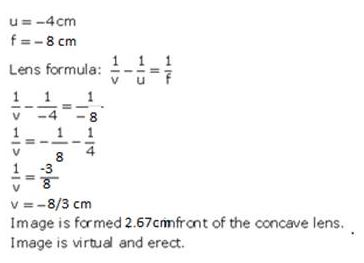9. A concave lens has a focal length of 20 cm. At what distance from the lens a 5 cm tall object is placed so that it forms an image at 10 cm from the lens? Also calculate the size of the image formed.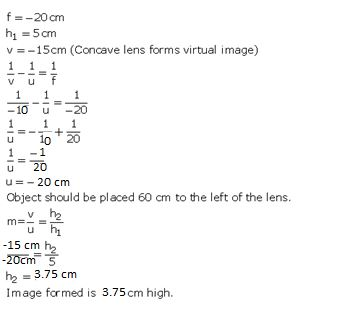10. An object is placed 20 cm from
(a) A converging lens, and
(b) A diverging lens, of focal length 15 cm. Calculate the image position and magnification in each case.11. A 2.0 cm tall object is placed 40 cm from a diverging lens of focal length 15 cm. Find the position and size of the image.12. Find the position and size of the virtual image formed when an object 2 cm tall is placed 20 cm from:
(i) a diverging lens of focal length 40 cm.
(ii) A converging lens of focal length 40 cm.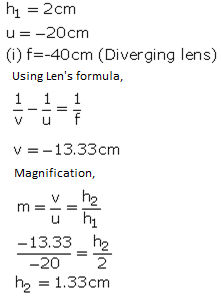13A. A small object is placed 150 mm away from a diverging lens of focal length 100 mm.
(i) Copy the figure below and draw rays to show how an image is formed by the lens.
(ii) Calculate the distance of the image from the lens by using the lens formula.

(i)(ii) object distance =150 mm
Focal length = 100 mm
Using len’s formula,
Image distance, v =- 60 mm

13B. The diverging lens in part (a) is replaced by a converging lens also of focal length 100 mm. The object remains in the same position and an image is formed by the converging lens. Compare two properties of this image with those of the image formed by the diverging lens in part (a).

→ Focal length = 100mm
Using len’s formula,
v = + 300 mm;
The image formed by converging lens is real, inverted and magnified (2 times). It is formed behind the converging lens. On the other hand, the image formed by diverging lens is virtual, erect and diminished. It is formed in front of the diverging lens.

Multiple Choice Questions (MCQs)-Pg-256

14. A concave lens produces an image 20 cm from the lens of an object placed 30 cm from the lens. The focal length of the lens is:
A. 50 cm
B. 40 cm
C. 60 cm
D. 30 cm15. Only one of the following applies to a concave lens. This is:
A. Focal length is positive
B. Image distance can be positive or negative
C. Height of image can be positive or negative
D. Image distance is always negative

→ Image is always formed in front of the mirror thus can not be negative.

16. The magnification produced by a spherical mirror and a spherical lens is + 0.8.
A. The mirror and lens are both convex
B. The mirror and lens are both concave
C. The mirror is concave but the lens is convex
D. The mirror is convex but the lens is concave

→ Concave lens and convex mirror forms a virtual and erect image.

17. The magnification produced by a spherical lens and a spherical mirror is+ 2.0.
A. The lens and mirror are both concave
B. The lens and mirror are both convex
C. The lens is convex but the mirror is concave
D. The lens is concave but the mirror is convex

→ Magnification of a convex and concave mirror is always positive.

Questions Based on High Order Thinking Skills (HOTS)-Pg-257

18. A camera fitted with a lens of focal length 50 mm is being used to photograph a flower that is 5 cm in diameter. The flower is placed 20 cm in front of the camera lens.
(a) At what distance from the film should the lens be adjusted to obtain a sharp image of the flower?
(b) What would be the diameter of the image of the flower on the film?
(c) What is the nature of camera lens?

→ (a) focal length, f = 50mm
object distance, u = -20 cm
Using len’s formula,
Image distance, v = + 6.66 cm ;
The film should be at a distance of 6.66 cm behind the camera lens
(b) the diameter of the image of the flower on the film is 1.66 cm
(c) the nature of camera lens is Convex lens.

19. An object is 2m from a lens which forms an erect image half (exactly) the size of the object. Determine the focal length of the lens. What type of lens is this?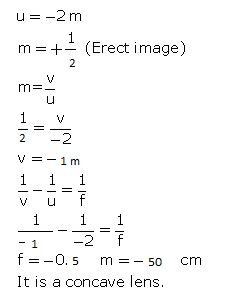20. An image formed on a screen is three times the size of the. Object. The object and screen are 80 cm apart when the image is sharply focused.
(a) State which type of lens is used.
(b) Calculate focal length of the lens.1. The lens A has a focal length of 25 cm whereas another lens B has a focal length of 60 cm. Giving reason state, which lens has more power : A or B.

→ Focal length of lens A = 25cm
Focal length of lens B = 60cm

Thus, Lens A has more power and It has shorter focal length.

2. Which causes more bending (or more refraction) of light rays passing through it : a convex lens of long focal length or a convex lens of short focal length ?

→ Convex lens of short focal length causes more bending (or more refraction) of light rays passing through it.

3. Name the physical quantity whose unit is dioptre.

→ Power of a lens has unit as dioptre.

4. Define 1 dioptre power of a lens.

→ 1 diopter is the power of a lens whose focal length is 1 metre.

5. Which type of lens has (a) a positive power, and (b) a negative power ?

→ a) Convex lens has Positive power.
b) Concave lens has Negative power.

6. Which of the two has a greater power: a lens of short focal length or a lens of large focal length ?

→ Lens of short focal length has a greater power.

7. How is the power of a lens related to its focal length?

→ Power of lens is reciprocal of its focal length in metres.
P = 1/f.

8. Which has more power : a thick convex lens or a thin convex lens, made of the same glass ? Give reason for your choice.

→ Thick convex lens has more power as It has shorter focal length.

9. The focal length of a convex lens is 50 cm. What is its power ?

→ Focal length = 50 cm = 0.50 m
P = 1/f = 1/0.50 = +2 D.

10. What is the power of a convex lens of focal length 0.25 m ?

→ f = 0.25 m
P = 1/f = 1/0.25 = +4 D.

11. A converging lens has a focal length of 50 mm. What is the power of the lens ?

→ focal length = 50 mm = 0.05 m
P = 1/f
= 1/0.05
= +20 D
Power is 20 Dioptre.

12. What is the power of a convex lens whole focal length is 80 cm ?

→ Focal length = 80 cm = 0.8 m
P = 1/f
= 1/0.8
= +1.25 D
Power is +1.25 Dioptre.

13. A diverging lens has a focal length of 3 cm. Calculate the power.

→ Here, f = -3 cm = -0.03 m (Diverging lens)
P = 1/f
= 1/(-0.03)
= -33.33 D
Power of the diverging lens is -33.33 Dioptre.

14. The power of a lens is + 0.2 D. Calculate its focal length.

→ P = +0.2 D
P = 1/f
f = 1/P = 1/0.2 = +5 m
Focal length = 5m.

15. The power of a lens is, - 2 D. What is its focal length ?

→ Power of a lens = -2 D
P = 1/f
f = 1/P = 1/(-2) = -0.5 m = -50 cm
Focal length is -50 cm.

16. What is the nature of a lens having a power of + 0.5 D ?

→ Nature of a lens having a power of + 0.5 D is Convex lens.

17. What is the nature of a lens whose power is,- 4 D?

→ The nature of a lens whose power is,- 4 D is Concave lens.

18. The optician's prescription for a spectacle lens is marked+ 0.5 D. What is the: (a) nature of spectacle lens?
(b) focal length of spectacle lens ?

→ (a) nature of spectacle lens is Convex lens
(b) Power of a lens = +0.5 D
P = 1/f
f = 1/P = 1/(+0.5) = +2 m
Focal length is 2 m.

19. A doctor has prescribed a corrective lens of power, -2 D. Find the focal length of the lens. Is the prescribed lens diverging or converging ?

→ P = -2 D
P = 1/f
f = 1/P = 1/(-2) = -0.5 m = -50 cm
Since focal length is negative, it is a diverging lens.

20. A lens has a focal length of, 10 cm. What is the power of the lens and what is its nature ?

→ f = 10 cm = 0.1 m
P = 1/f = 1/(0.1) = 10 D
It is a convex lens.

21. The focal length of a lens is +150 mm. What kind of lens is it and what is its power ?

→ f = 150 mm = 0.15 m
P = 1/f = 1/0.15 = +6.6.D
kind of lens is Convex lens ; its power is + 6.6 D.

22. Fill in the following blanks with suitable words :
(a) The reciprocal of the focal length in ____ gives you the power of the lens, which is measured in........
(b) For _____ lenses, the power is positive while for diverging lenses, the power is negative

→ (a) metres; dioptres
(b) converging, diverging.

23. An object of height 4 cm is placed at a distance of 15 cm in front of a concave lens of power, -10 dioptres. Find the size of the image.24. An object of height 4.25 mm is placed at a distance of 10 cm from a convex lens of power +5 D. Find (i) foe length of the lens, and (ii) size of the image.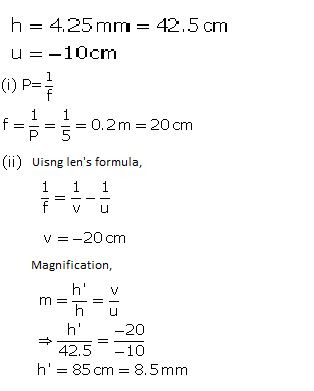25. A convex lens of power S D and a concave lens of power 7.5 D are placed in contact with each other. What is the:
(a) power of this combination of lenses?
(b) focal length of this combination of lenses ?

→ Power of convex lens = 5 D
Power of concave lens = -7.5 D
(a) Power of the combination of the lens = P1+ P2 = 5 – 7.5= -2.5 D
(b) P = 1/f
f = 1/P = 1/ -2.5 = -40 cm.

26. A convex lens of focal length 2S cm and a concave lens of focal length 10 cm are placed in close contact with one another.
(a) What is the power of this combination?
(b) What is the focal length of this combination?
(c) Is this combination converging or diverging ?

→ Focal length of convex lens= 25 cm = 0.25 m
Power = 1/f = 1/0.25 = + 4 D
Focal length of concave lens = -10 cm = -0.1 m
Power = 1/f = -1/0.1 = - 10 D
(a) Power of combination = P1+ P2 = 4 D – 10 D = -6 D
(b) f = 1/ P = 1/ -6 = -16.66 cm
(c) This combination is Diverging.

27. The power of a combination of two lenses X and Y is S D. If the focal length of lens X be 1S cm :
(a) calculate the focal length of lens Y.
(b) state the nature of lens Y.

→ Power of combination = 5 D
Focal length of X = 15 cm= 0.15 m
Power of X = 1/ 0.15 = 6.67 D
(a) Power of Y = 5 – 6.67 = -1.67 D
f = 1/P = - 60 cm
(b) Nature of lens is Concave lens.

28. Two lenses A and B have focal lengths of+ 20 cm and, -10 cm, respectively.
(a) What is the nature of lens A and lens B?
(b) What is the power of lens A and lens B?
(c) What is the power of combination if lenses A and B are held close together?

→ Focal length of lens A = +20 cm = 0.20m
Power of lens A = 1/f = 1/0.20 = +5D
Focal length of lens B = - 10 cm = -0.1 m
Power of lens B = 1/f = 1/-0.1 = -10 D
(a) Lens A is convex and Lens B is concave
(b) Power of lens A = + 5 D; Power of lens B = -10 D
(c) power of combination = P1+ P2 = 5 D -10D = -5D.

29A. What do you understand by the power of a lens ? Name one factor on which the power of a lens depend.

→ Power of lens is reciprocal of its focal length in metres.
P = 1/f
It depends on the focal length of the lens.

29B. What is the unit of power of a lens? Define the unit of power of a lens.

→ Unit of lens is dioptre (D).

29C. A combination of lenses for a camera contains two converging lenses of focal lengths 20 cm and 40 cm and a diverging lens of focal length 50 cm. Find the power and focal length of the combination.

Focal length of 1 lens = 20 cm = 0.2 m
Power 1 = 1/ 0.2 = +5 D
Focal length of 2nd lens = 40 cm = 0.4 m
Power 2 = 1/ 0.4 = 0.25 D
Power = P1 + P2 = +5D + 0.25 D = + 5.25 D ;
P = 1/f
f = + 18.18 cm.

30A. Two lenses A and B have power of (i) + 2 D and (ii) - 4 D respectively. What is the nature and f length of each lens ?

→ Power of lens A = +2D
Power of lens B = -4 D
Lens A is convex; f =+50 cm; Lens B is concave ; f =- 25 cm.

30B. An object is placed at a distance of 100 cm ,from each of the above lenses A and B. Calculate (i) image distance, and (ii) magnification, in each of the two cases.

→ Lens A : v = +100 cm ; m = - 1 ; Lens B : v = -20 cm ; m = 0.2.

Multiple Choice Questions (MCQs)-Pg-262

31. The focal lengths of four convex lenses P, Q, R and S are 20 cm, 15 cm, 5 cm and 10 cm, respectively. lens having greatest power is:
A. P
B. Q
C. R
D. S

→ P = 1/f.

32. A converging lens has a focal length of 50 cm. The power of this lens is:
A. + 0.2 D
B. - 2.0 D
C. + 2.0 D
D. - 0.2 D

→ P = 1/f (m).

33. A diverging lens has a focal length of 0.10 m. The power of this lens will be:
A. + 10.0 D
B. + 1.0 D
C. - 1.0 D
D. - 10.0 D

→ P = 1/f (m).

34. The power of a lens is + 2.0 D. Its focal length should be :
A. 100 cm
B. 50 cm
C. 25 cm
D. 40 cm

→ P = 1/f (m).

35. If a spherical lens has a power of,- 0.25 D, the focal length of this lens will be:
A. - 4 cm
B. - 400 mm
C. - 4 m
D. - 40 m

→ P = 1/f (m).

36. The power of a concave lens is 10 D and that of a convex lens is 6 D. When these two lenses are placed in contact with each other, the power of their combination will be :
A. + 16 D
B. + 4 D
C.-16 D
D. - 4 D

→ P = 1/f (m).

37. The power of a converging lens is 4.5 D and that of a diverging lens is 3 D. The power of this combination of lenses placed close together is :
A. + 1.5 D
B. + 7.5 D
C. - 7.5 D
D. - 1.5 D

→ P = 1/f (m).

38. A convex lens of focal length 10 cm is placed in contact with a concave lens of focal length 20 cm. The focal length of this combination of lenses will be :
A. + 10 cm
B. + 20 cm
C. - 10 cm
D. - 20 cm

→ P = 1/f (m)
P = P1 + P2 + P3 …………..

Questions Based on High Order Thinking Skills (HOTS)-Pg-263

39. The optical prescription for a pair of spectacles is:
Right eye: - 3.50 D Left eye: - 4.00 D
(a) Are these lenses thinner at the middle or at the edges?
(b) Which lens has a greater focal length?
(c) Which is the weaker eye?

→ Right eye : - 3.5 D
→ Left eye : -4 D.

40. A person got his eyes tested by an optician. The prescription for the spectacle lenses to be made reads:
Left eye: + 2.50 D Right eye: + 2.00 D
(a) State whether these lenses are thicker in the middle or at the edges.
(b) Which lens bends the light rays more strongly?
(c) State whether these spectacle lenses will converge light rays or diverge light rays.